Open In App

# CBSE Class 10 Term 1 Science Answer Key 2021-22

The CBSE Class 10th Term 1 Science Exam was conducted by the Central Board of Secondary Education on December 2, 2021, Thursday. This was the second major paper of Class 10 after Social Science. The examination was conducted in over 25000 exam centres across the country.

CBSE Class 10th Term 1 Science Exam was MCQ based paper for 90 minutes, in which students need to attempt a total of 50 questions. In total, the question paper consists of 60 multiple choice type questions (MCQs). Students were required to attempt 50 questions. MCQs of different formats like questions based on diagrams, graphs, case study, assertion reason and competency were there in the paper.

The exam was application based and tested the thinking skills of the students. There were a number of competency-based questions.

CBSE Class 10 Science question paper 2021 was of a good standard and thought-provoking. Equal emphasis was given to activities, numerical and diagram based interpretation questions. Few questions required in-depth reading and interpretation. All questions were within the syllabus.

The difficulty level was average. All students appeared comfortable while attempting the exam and were satisfied with the overall experience.

Questions were balanced and the paper could be completed on time. The paper tested the logical thinking skills of students and in-depth knowledge of the NCERT was required. The paper followed the pattern set by the sample paper set by CBSE. Some experts call the Science paper average.

Questions in the paper were entirely from the syllabus. The reason for teachers and experts calling it an average/moderate paper is that some of the questions were asked indirectly which could have confused the students. However, one with in-depth knowledge of the concepts and those who had grasped the NCERT thoroughly would have answered all the questions easily.

GeeksforGeeks exclusively presents the CBSE Answer Key of the Class 10 Science Term 1 Question Paper. Students can refer to this answer key prepared by the subject experts to cross-check their answers and get an idea of the expected marks in their Science Exam 2021-22.

### Term- I

Time allowed- 90 minutes

Maximum Marks- 40

General Instructions

1. This question paper contains 60 questions out of which 50 questions are to be attempted. All questions carry equal marks.
2. The question paper consists of three Sections – Section A, B and C.
3. Section – A contains 24 questions. Attempt any 20 questions from Question number 1 to 24.
4. Section – B also contains 24 questions. Attempt any 20 questions from Question number 25 to 48.
5. Section – C contains three Case Studies containing 4 questions in each case. Attempt any 10 questions from Question number 49 to 60.
6. There is only one correct option for every Multiple Choice Question (MCQ). Marks will not be awarded for answering more than one option.
7. There is no negative marking.

### SECTION – A

Question Numbers 1 to 24 are of 1 mark each. Any 20 Questions from Question 1 to 24 are needed to be attempted.

Question 1: A student took Sodium Sulphate solution in a test tube and added Barium Chloride solution to it. He observed that an insoluble substance has formed. The colour and molecular formula of the insoluble substance is

(a) Grey, Ba2SO4

(b) Yellow, Ba(SO4)2

(c) White, BaSO4

(d) Pink, BaSO4

Question 2: Which of the following oxide(s) is/are soluble in water to form alkalis?

(i) Na2O            (ii) SO2            (iii) K2O            (iv) NO2

(a) (i) and (iii)

(b) (i) only

(c) (ii) and (iv)

(d) (iii) only

Question 3: Study the diagram given below and identify the gas formed in the reaction.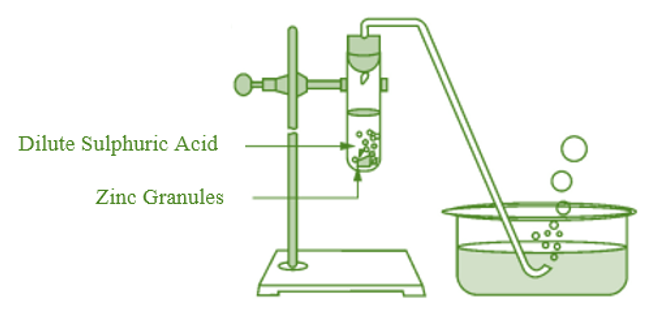(a) Carbon di-oxide which extinguishes the burning candle.

(b) Oxygen due to which the candle burns more brightly.

(c) Sulphur dioxide which produces a suffocating smell.

(d) Hydrogen which while burning produces a popping sound.

Question 4: Sodium reacts with water to form sodium hydroxide and hydrogen gas. The balanced equation which represents the above reaction is

(a) Na(s) + 2H2O(l) → 2NaOH(aq) + 2H2(g)

(b) 2Na(s) + 2H2O(l) → 2NaOH(aq) +H2(g)

(c) 2Na(s) + 2H2O(l) → NaOH(aq) + 2H2(g)

(d) 2Na(s) + H2O(l) – 2NaOH(aq) + 2H2(g)

Question 5: Which of the options in the given table are correct?

(a) (i) and (ii)
(b) (i) and (iv)
(c) (ii) and (iii)
(d) (ii) and (iv)

Question 6: C6H12O6 (aq) + 6O2 (aq) ⇢ 6CO2 (aq) + 6H2O (l)

The above reaction is a/an

(a) displacement reaction

(b) endothermic reaction

(c) exothermic reaction

(d) neutralisation reaction

Question 7: Which of the following statements about the reaction given below are correct?

MnO2 + 4HCl ⇢ MnCl2 + 2H2O + Cl2

(i) HCl is oxidized to Cl2

(ii) MnO2 is reduced to MnCl2

(iii) MnCl2 acts as an oxidizing agent

(iv) HCl acts as on oxidizing agent

(a) (ii), (iii) and (iv)

(b) (i), (ii) and (iii)

(c) (i) and (ii) only

(d) (iii) and (iv) only

Question 8: Select from the following the statement which is true for bases.

(a) Bases are bitter and turn blue litmus red.

(b) Bases have a pH less than 7.

(c) Bases are sour and change red litmus to blue.

(d) Bases turn pink when a drop of phenolphthalein is added to them.

Question 9: Study the following table and choose the correct option:

Question 10: It is important to balance the chemical equations to satisfy the law of conservation of mass. Which of the following statements of the law is incorrect?

(a) The total mass of the elements present in the reactants is equal to the total mass of the elements presents in the products.

(b) The number of atoms of each element remains the same, before and after a chemical reaction.

(c) The chemical composition of the reactants is the same before and after the reaction.

(d) Mass can neither be created nor can it be destroyed in a chemical reaction.

Question 11: Consider the following statements in connection with the functions of the blood vessels marked A and B in the diagram of a human heart as shown.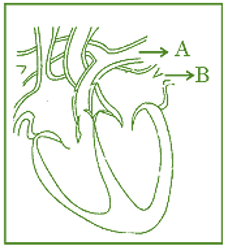(i) Blood vessel A – It carries carbon dioxide-rich blood to the lungs.

(ii) Blood vessel B – It carries oxygen-rich blood from the lungs.

(iii) Blood vessel B – Left atrium relaxes as it receives blood from this blood vessel

(iv) Blood vessel A – Right atrium has a thick muscular wall as it has to pump blood to this blood vessel.

The correct statements are:

(a) (i) and (ii) only

(b) (ii) and (iii) only

(c) (ii), (iii) and (iv)

(d) (i), (ii) and (iii)

Question 12: In living organisms during respiration which of the following products are not formed if oxygen is not available?

(a) Carbon dioxide + Water

(b) Carbon dioxide + Alcohol

(c) Lactic acid + Alcohol

(d) Carbon dioxide + Lactic Acid

Question 13: The correct statements with reference to single-celled organisms are

(i) Complex substances are not broken down into simpler substances.

(ii) Simple diffusion is sufficient to meet the requirement of the exchange of gases.

(iii) Specialised tissues perform different functions in the organism.

(iv) Entire surface of the organism is in contact with the environment for taking in food.

(a) (i) and (iii)

(b) (ii) and (iii)

(c) (ii) and (iv)

(d) (i) and (iv)

Question 14: Which one among the following is not removed as a waste product from the body of a plant?

(a) Resins and Gums

(b) Urea

(c) Dry Leaves

(d) Excess Water

Question 15: Which of the following statements are correct in reference to the role of A (shown in the given diagram) during a breathing cycle in human beings?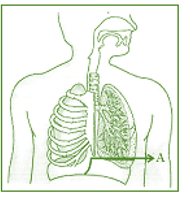(i) It helps to decrease the residual volume of air in the lungs.

(ii) It flattens as we inhale.

(iii) It gets raised as we inhale.

(iv) It helps the chest cavity to become larger.

(a) (ii) and (iv)

(b) (iii) and (iv)

(c) (i) and (ii)

(d) (i), (ii) and (iv)

Question 16: Which one of the following conditions is true for the state of stomata of a green leaf is shown in the given diagram?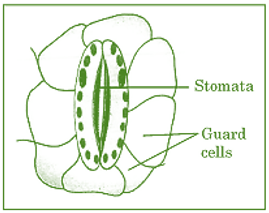(a) Large amount of water flows into the guard cells.

(b) Gaseous exchange is occurring in a large amount.

(c) Large amount of water flows out from the guard cells.

(d) Large amount of sugar collects in the guard cells.

Question 17: In which of the following is a concave mirror used?

(a) A solar cooker

(b) A rearview mirror in vehicles

(c) A safety mirror in shopping malls

(d) In viewing full-size images of distant tall buildings.

Question 18: A student wants to obtain a magnified image of an object AB as on a screen. Which one of the following arrangements shows the correct position of AB for him/her to be successful?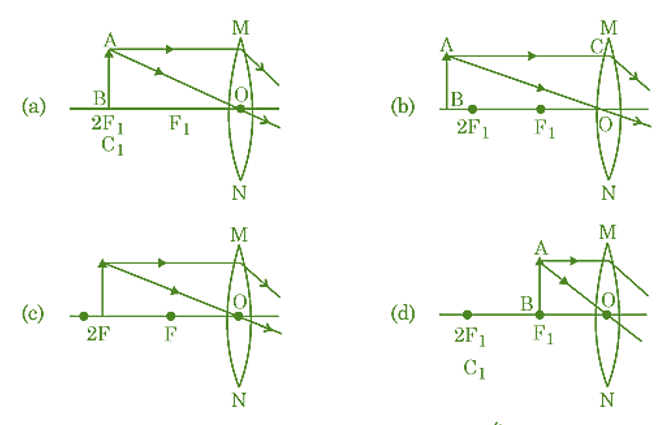Question 19: The following diagram shows the use of an optical device to perform an experiment of light. As per the arrangement shown, the optical device is likely to be a;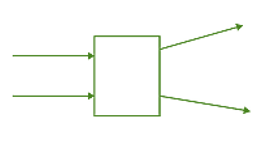(a) Concave mirror

(b) Concave lens

(c) Convex mirror

(d) Convex lens

Question 20: A ray of light starting from the air passes through medium A of refractive index 1.50, enters medium B of refractive index 1.33 and finally enters medium C of refractive index 2.42. If this ray emerges out in the air from C, then for which of the following pairs of media is the bending of light is least?

(a) air-A

(b) A-B

(c) B-C

(d) C-air

Question 21: Which of the following statements is not true for the scattering of light?

(a) Colour of the scattered light depends on the size of particles of the atmosphere.

(b) Red light is least scattered in the atmosphere.

(c) Scattering of light takes place as various colours of white light travel with different speeds in air.

(d) The fine particles in the atmospheric air scatter the blue light more strongly than red. So the scattered blue light enters our eyes.

Question 22: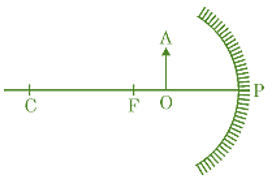For the diagram shown, according to the new Cartesian sign convention the magnification of the image formed will have the following specifications :

(a) Sign – Positive, Value – Less than 1

(b) Sign – Positive, Value – More than 1

(c) Sign – Negative, Value – Less than 1

(d) Sign – Negative, Value – More than 1

Question 23: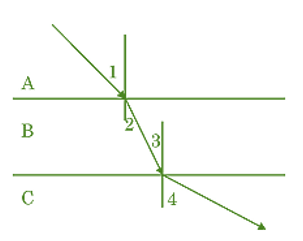A ray of light is incident as shown. If A, B and C are three different transparent media, then which among the following options is true for the given diagram?

(a) ∠ 1 > ∠ 4

(b) ∠ 1 < ∠ 2

(c) ∠ 3 = ∠ 2

(d) ∠ 3 > ∠ 4

Question 24: In the diagram given below, X and Y are the end colours of the spectrum of white light. The colour of ‘Y’ represents the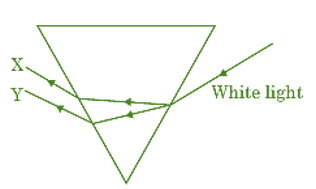(a) Colour of the sky as seen from Earth during the day.

(b) Colour of the sky as seen from the moon.

(c) Colour used to paint the danger signals.

(d) Colour of the sun at the time of noon

### SECTION – B

This Section consists of total of 24 questions i.e. from Question number 25 to 48. Out of which 20 questions are needed to be attempted from this section.

Question 25: Which one of the following reactions is categorised as a thermal decomposition reaction?

(a) 2H2O (l) ⇢ 2H2 (g) + O2 (g)

(b) 2AgBr(s) ⇢ 2Ag(s) + Br2 (g)

(c) 2AgCl (s) ⇢ 2Ag(s) + Cl2 (g)

(d) CaCO3 (s) ⇢ CaO(s) + CO2 (g)

Question 26: Consider the pH value of the following acidic samples :

The decreasing order of their H+ ion concentration is

(a) 3 > 4 > 1 > 2

(b) 2 > 1 > 3 > 4

(c) 2 > 1 > 4 > 3

(d) 3 > 4 > 2 > 1

Question 27: Study the experimental set-up shown in the given figure and choose the correct option from the following: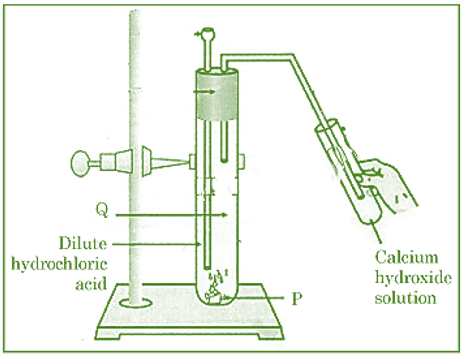Question 28: Which one of the following structures correctly depicts the compound CaCl2?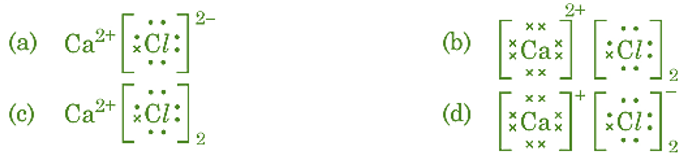Question 29: The pair(s) which will show displacement reaction is/are

(i) NaCl solution and copper metal

(ii) AgNO3 solution and copper metal

(iii) Al2(SO4)3 solution and magnesium metal

(iv) ZnSO4 solution and iron metal

(a) (ii) only

(b) (ii) and (iii)

(c) (iii) and (iv)

(d) (i) and (ii)

Question 30: Which of the following salts do not have the water of crystallisation?

(i) Bleaching Powder

(ii) Plaster of Paris

(iii) Washing soda

(iv) Baking soda

(a) (ii) and (iv)

(b) (i) and (iii)

(c) (ii) and (iii)

(d) (i) and (iv)

Question Number 31-35 consists of two statements – Assertion (A) and Reason (R).

Answer these questions selecting the appropriate option given below:

(a) Both (A) and (R) are true and (R) is the correct explanation of (A).

(b) Both (A) and (R) are true but (R) is not the correct explanation of (A).

(c) (A) is true, but (R) is false.

(d) (A) is false, but (R) is true.

Question 31: Assertion (A): Sodium hydrogen carbonate is used as an ingredient in antacids.

Reason (R): NaHCO3 is a mild non-corrosive basic salt.

Question 32: Assertion (A): Burning of Natural gas is an endothermic process.

Reason (R): Methane gas combines with oxygen to produce carbon dioxide and water.

Question 33: Assertion (A): Nitrogen is an essential element for plant growth and is

taken up by plants in the form of inorganic nitrates or nitrites.

Reason (R): The soil is the nearest and richest source of raw materials like Nitrogen, Phosphorus and other minerals for the plants.

Question 34: Assertion (A): Sun appears reddish at the time of Sunrise and Sunset.

Reason (R): Distance travelled by sunlight in the atmosphere is lesser during sunrise and sunset as compared to noon.

Question 35: Assertion (A): Hydrochloric acid helps in the digestion of food in the stomach.

Reason (R): Hydrochloric acid creates an acidic medium to activate protein-digesting enzymes.

Question 36: A student was asked to write a stepwise procedure to demonstrate that carbon dioxide is necessary for photosynthesis. He wrote the following steps. The wrongly worded step is –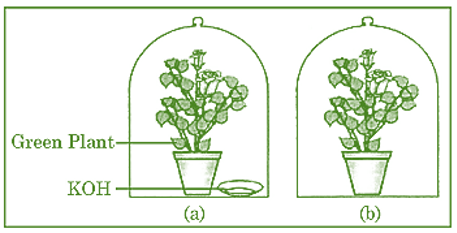(a) Both potted plants are kept in a dark room for at least three days.

(b) Bottom of the bell jars is sealed to make them airtight.

(c) Both potted plants are kept in sunlight after the starch test.

(d) A leaf from both the plants is taken to test the presence of starch.

Question 37: Respiratory structures of two different animals-a fish and a human being are as shown. Observe (a) and (b) and select one characteristic that holds true for both of them.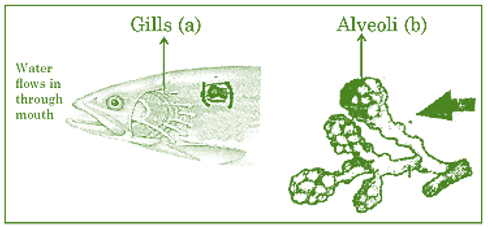(a) Both are placed internally in the body of an animal.

(b) Both have thin and moist surfaces for gaseous exchange.

(c) Both are poorly supplied with blood vessels to conserve energy.

(d) In both the blood returns to the heart after being oxygenated.

Question 38: Observe the diagram of an activity given below. What does it help to conclude, when the person exhales into the test tube?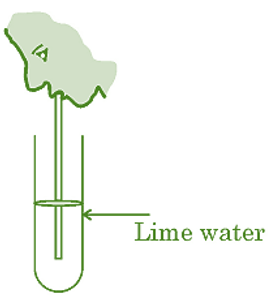(a) Percentage of carbon dioxide is more in inhaled air.

(b) Fermentation occurs in the presence of oxygen.

(c) Percentage of carbon dioxide is more in the exhaled air.

(d) Fermentation occurs in the presence of carbon dioxide.

Question 39: If a lens can converge the sun rays at a point 20 cm. away from its optical centre, the power of this lens is –

(a) + 2D

(b) – 2D

(c) + 5D

(d) – 5D

Question 40: The radius of curvature of a converging mirror is 30 cm. At what distance from the mirror should an object be placed so as to obtain a virtual image?

(a) Infinity

(b) 30 cm

(c) Between 15 cm and 30 cm

(d) Between 0 cm and 15 cm

Question 41: The length of the small intestine in a deer is more as compared to the length of the small intestine of a tiger. The reason for this is –

(a) Mode of intake of food.

(b) Type of food consumed.

(c) Presence or absence of villi in intestines.

(d) Presence or absence of digestive enzymes.

Question 42: Identify the two components of Phloem tissue that help in the transportation of food in plants.

(a) Phloem parenchyma & sieve tubes

(b) Sieve tubes & companion cells

(c) Phloem parenchyma & companion cells

(d) Phloem fibres and sieve tubes

Question 43: A converging lens forms a three-times magnified image of an object, which can be taken on a screen. If the focal length of the lens is 30 cm, then the distance of the object from the lens is

(a) – 55 cm

(b) – 50 cm

(c) – 45 cm

(d) – 40 cm

Question 44: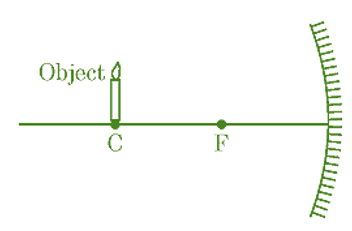Which of the following statements is not true in reference to the diagram shown above?

(a) Image formed is real.

(b) Image formed is enlarged.

(c) Image is formed at a distance equal to double the focal length.

(d) Image formed is inverted.

Question 45: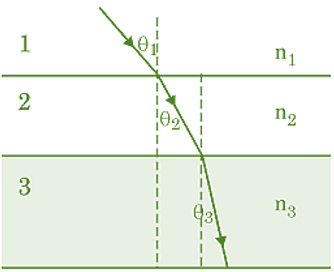In the diagram shown above n1, n2 and n3 are refractive indices of the media 1, 2 and 3 respectively. Which one of the following is true in this case?

(a) n1 = n2

(b) n1 > n2

(c) n2 > n3

(d) n3 > n1

Question 46: The refractive index of medium A is 1.5 and that of medium B is 1.33. If the speed of light in the air is 3 × 108 m/s, what is the speed of light in medium A and B respectively?

(a) 2 × 108 m/s and 1.33 × 108 m/s

(b) 1.33 × 108 m/s and 2 × 108 m/s

(c) 2.25 × 108 m/s and 2 × 108 m/s

(d) 2 × 108 m/s and 2.25 × 108 m/s

Question 47: An object of height 4 cm is kept at a distance of 30 cm from the pole of a diverging mirror. If the focal length of the mirror is 10 cm, the height of the image formed is

(a) + 3.0 cm

(b) + 2.5 cm

(c) + 1.0 cm

(d) + 0.75 cm

Question 48: 50.0 mL of tap water was taken in a beaker. Hydrochloric acid was added drop by drop to water. The temperature and pH of the solution was noted. The following graph was obtained. Choose the correct statements related to this activity.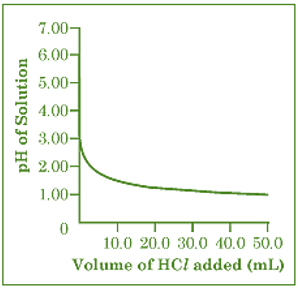(i) The process of dissolving an acid in water is highly endothermic.

(ii) The pH of the solution increases rapidly with the addition of acid.

(iii) The pH of the solution decreases rapidly with the addition of acid.

(iv) The pH of tap water was around 7.0.

(a) (i) and (ii)

(b) (i) and (iii)

(c) (iii) and (iv)

(d) (ii) and (iv)

### SECTION – C

This Section consists of three cases followed by questions. There are a total of 12 questions (Q. No. 49 to 60) in this section. Attempt any 10 questions from this section. The first attempted 10 questions would be evaluated.

Case-I:

A student, took four metals P, Q, R and S and carried out different experiments to study the properties of metals. Some of the observations were :

• All metals could not be cut with a knife except metal R.
• Metal P combined with oxygen to form an oxide M2O3 which reacted with both acids and bases.
• Reaction with water.

P – Did not react either with cold or hot water but reacted with steam

Q – Reacted with hot water and the metal started floating

R – Reacted violently with cold water.

S – Did not react with water at all

Based on the above observations answer the following:

Question 49: Out of the given metals, the one which needs to be stored used Kerosene is

(a) P

(b) R

(c) S

(d) Q

Question 50: Out of the given metals, the metal Q is

(a) Iron

(b) Zinc

(c) Potassium

(d) Magnesium

Question 51: The metal which forms amphoteric oxides is

(a) P

(b) Q

(c) R

(d) S

Question 52: The increasing order of the reactivity of the four metals is;

(a) P < Q < R < S

(b) S < R < Q < P

(c) S < P < Q < R

(d) P < R < Q < S

Case-II:

The figure shown below represents a common type of dialysis called as Haemodialysis. It removes waste products from the blood. Such as excess salts, and urea which are insufficiently removed by the kidney in patients with kidney failure. During the procedure, the patient’s blood is cleaned by filtration through a series of semi-permeable membranes before being returned to the blood of the patient. On the basis of this, answer the following questions: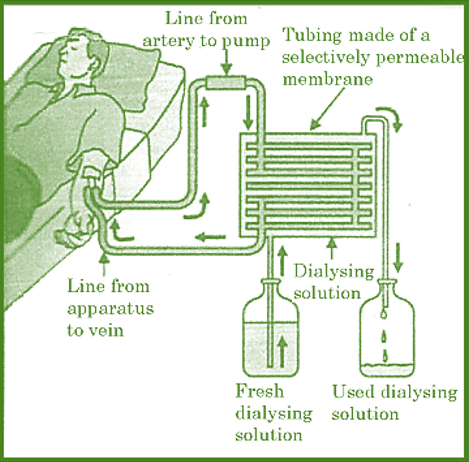Question 53: The haemodialyzer has a semi-permeable lining of tubes which help to:

(a) To maintain the osmotic pressure of blood.

(b) To filter nitrogenous wastes from the dialyzing solution.

(c) In passing the waste products in the dialyzing solution.

(d) To pump purified blood back into the body of the patient.

Question 54: Which one of the following is not a function of the Artificial Kidney?

(a) To remove nitrogenous wastes from the blood.

(b) To remove excess fluids from the blood.

(c) To reabsorb essential nutrients from the blood.

(d) To filter and purify the blood.

Question 55: The ‘used dialysing’ solution is rich in;

(a) Urea and excess salts

(b) Blood cells

(c) Lymph

(d) Proteins

Question 56: Which part of the nephron in the human kidney, serves the function of reabsorption of certain substances?

(a) Glomerulus

(b) Bowmans Capsule

(c) Tubules

(d) Collecting duct

Case-III:

A compound microscope is an instrument that consists of two lenses L1 and L2. The lens L1 called objective forms a real, inverted and magnified image of the given object. This serves as the object for the second lens L2; the eyepiece. The eyepiece functions as a simple microscope or magnifier. It produces the final image, which is inverted with respect to the original object, enlarged and virtual.

Question 57: What types of lenses must be L1 and L2?

(a) Both concave

(b) Both convex

(c) L1 – concave and L2 – convex

(d) L1 – convex and L2 – concave

Question 58: What is the value and sign of magnification (according to the new Cartesian sign convention) of the image formed by L1?

(a) Value = Less than 1 and Sign = Positive

(b) Value = More than 1 and Sign = Positive

(c) Value = Less than 1 and Sign = Negative

(d) Value = More than 1 and Sign = Negative

Question 59: What is the value and sign of (according to the new Cartesian sign convention) magnification of the image formed by L2?

(a) Value = Less than 1 and Sign = Positive

(b) Value = More than 1 and Sign = Positive

(c) Value = Less than 1 and Sign = Negative

(d) Value = More than 1 and Sign = Negative